mscroggs.co.uk
mscroggs.co.uksubscribe

# Blog

2020-07-29
A week ago, it was 22 July: Pi Approximation Day. 22/7 (22 July in DD/M format) is very close to pi, closer in fact than 14 March's approximation of 3.14 (M.DD).
During this year's Pi Approximation Day, I was wondering if there are other days that give good approximations of interesting numbers. In particular, I wondered if there is a good 2π (or τ) approximation day.
π is close to 22/7, so 2π is close to 44/7—but sadly there is no 44th July. The best approximation day for 2π is 25th April, but 25/4 (6.25) isn't really close to 2π (6.283185...) at all. The day after Pi Approximation Day, however, is a good approximation of 2π-3 (as π-3 is approximately 1/7). After noticing this, I realised that the next day would be a good approximation of 3π-6, giving a nice run of days in July that closely approximate expressions involving pi.
After I tweeted about these three, Peter Rowlett suggested that I could get a Twitter bot to do the work for me. So I made one: @HappyApproxDay.
@HappyApproxDay is currently looking for days that approximate expressions involving π, τ, e, √2 and √3, and approximate the chosen expression better than any other day of the year. There are an awful lot of ways to combine these numbers, so @HappyApproxDay looks like it might be tweeting quite a lot...

### Similar postsLogic bot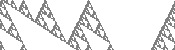Close encounters of the second kind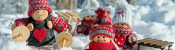Christmas (2020) is over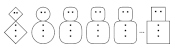Christmas card 2020

Comments in green were written by me. Comments in blue were not written by me.

Allowed HTML tags: <br> <a> <small> <b> <i> <s> <sup> <sub> <u> <spoiler> <ul> <ol> <li>
To prove you are not a spam bot, please type "a" then "x" then "e" then "s" in the box below (case sensitive):
2014-11-26
Last week, mathslogicbot started the long task of tweeting every tautology in propositional calculus. This post explains what this means and how I did it.

### What is propositional calculus?

Propositional calculus is a form of mathematical logic, in which the formulae (the logical 'sentences') are made up of the following symbols:
• Variables (a to z and $$\alpha$$ to $$\lambda$$) (Variables are usually written as $$p_1$$, $$p_2$$, etc. but as Twitter cannot display subscripts, I chose to use letters instead.)
• Not ($$\neg$$)
• Implies ($$\rightarrow$$)
• If and only if ($$\leftrightarrow$$)
• And ($$\wedge$$)
• Or ($$\vee$$)
• Brackets ($$()$$)

#### Formulae

Formulae are defined recursively using the following rules:
• Every variable is a formula.
• If $$A$$ is a formula, then $$\neg A$$ is a formula.
• If $$A$$ and $$B$$ are formulae then $$(A\rightarrow B)$$, $$(A\leftrightarrow B)$$, $$(A\wedge B)$$ and $$(A\vee B)$$ are all formulae.
For example, $$(a\vee b)$$, $$\neg f$$ and $$((a\vee b)\rightarrow\neg f)$$ are formulae.
Each of the variables is assigned a value of either "true" or "false", which leads to each formula being either true or false:
• $$\neg a$$ is true if $$a$$ is false (and false otherwise).
• $$(a\wedge b)$$ is true if $$a$$ and $$b$$ are both true (and false otherwise).
• $$(a\vee b)$$ is true if $$a$$ or $$b$$ is true (or both are true) (and false otherwise).
• $$(a\leftrightarrow b)$$ is true if $$a$$ and $$b$$ are either both true or both false (and false otherwise).
• ($$a \rightarrow\ b)$$ is true if $$a$$ and $$b$$ are both true or $$a$$ is false (and false otherwise).

#### Tautologies

A tautology is a formula that is true for any assigment of truth values to the variables. For example:
$$(a\vee \neg a)$$ is a tautology because: if $$a$$ is true then $$a$$ or $$\neg a$$ is true; and if $$a$$ is false, then $$\neg a$$ is true, so $$a$$ or $$\neg a$$ is true.
$$(a\leftrightarrow a)$$ is a tautology because: if $$a$$ is true then $$a$$ and $$a$$ are both true; and if $$a$$ is false then $$a$$ and $$a$$ are both false.
$$(a\wedge b)$$ is not a tautology because if $$a$$ is true and $$b$$ is false, then it is false.
The following are a few more tautologies. Can you explain why they are always true?
• $$(a\leftrightarrow a)$$
• $$((a\vee\neg a)\vee a)$$
• $$\neg(a\wedge\neg a)$$
• $$(a\vee(a\rightarrow b))$$

### Python

If you want to play with the Logic Bot code, you can download it here.
In order to find all tautologies less than 140 characters long, one method is to first generate all formulae less than 140 characters then check to see if they are tautologies. (This is almost certainly not the fastest way to do this, but as long as it generates tautolgies faster than I want to tweet them, it doesn't matter how fast it runs.) I am doing this on a Raspberry Pi using Python in the following way.

#### All formulae

The following code is writing all the formulae that are less than 140 characters to a file called formulae.
python
from os.path import join
path = "/home/pi/logic"
First import any modules needed and set the path where the file will be saved.
python
def candidate(formula):
global formulae

if len(formula) <= 140 and formula not in formulae:
formulae.append(formula)
print formula
f = open(join(path,"formulae"),"a")
f.write(formula + "\n")
f.close()
This function checks that a formula is not already in my list of formulae and shorter than 140 characters, then adds it to the list and writes it into the file.
python
variables = ["a""b""c""d""e""f""g""h""i""j",
"k""l""m""n""o""p""q""r""s""t",
"u""v""w""x""y""z""@""#""2""3",
"4""5""6""7""8""9"]
This line says which characters are going to be used as variables. It is impossible to write a formula in less that 140 characters with more than 36 different variables so these will be sufficient. I haven't used 0 and 1 as these are used to represent false and true later.
python
= open(join(path,"formulae"))
for i in range(0,len(formulae)):
formulae[i] = formulae[i].strip("\n")
f.close()
These lines load the formulae already found from the file. This is needed if I have to stop the code then want to continue.
python
oldlen = 0
newlen = 26

while oldlen != newlen:
for f in formulae + variables:
candidate("-" + f)
for f in formulae + variables:
for g in formulae:
for star in ["I""F""N""U"]:
candidate("(" + f + star + g + ")")
oldlen = newlen
newlen = len(formulae)
The code inside the while loop goes through every formula already found and puts "-" in front of it, then takes every pair of formulae already found and puts "I", "F", "N" or "U" between them. These characters are used instead of the logical symbols as using the unicode characters leads to numerous python errors. The candidate function as defined above then adds them to the list (if they are suitable). This continues until the loop does not make the list of formulae longer as this will occur when all formulae are found.
python
= open(join(path,"formulae"),"a")
f.write("#FINISHED#")
f.close()
Once the loop has finished this will add the string "#FINISHED#" to the file. This will tell the truth-checking code when the it has checked all the formulae (opposed to having checked all those generated so far).

#### Tautologies

Now that the above code is finding all formulae, I need to test which of these are tautologies. This can be done by checking whether every assignment of truth values to the variables will lead to the statement being true.
python
from os.path import join
path = "/home/pi/logic"
First import any modules needed and set the path where the file will be saved.
python
def next(ar,i=0):
global cont
if i < len(ar):
if ar[i] == "0":
ar[i] = "1"
else:
ar[i] = "0"
ar = next(ar, i + 1)
else:
cont = False
return ar
Given an assignment of truth values, this function will return the next assignment, setting cont to False if all the assignments have been tried.
python
def solve(lo):
lo = lo.replace("-0""1")
lo = lo.replace("-1""0")

lo = lo.replace("(0I0)""1")
lo = lo.replace("(0I1)""1")
lo = lo.replace("(1I0)""0")
lo = lo.replace("(1I1)""1")

lo = lo.replace("(0F0)""1")
lo = lo.replace("(0F1)""0")
lo = lo.replace("(1F0)""0")
lo = lo.replace("(1F1)""1")

lo = lo.replace("(0N0)""0")
lo = lo.replace("(0N1)""0")
lo = lo.replace("(1N0)""0")
lo = lo.replace("(1N1)""1")

lo = lo.replace("(0U0)""0")
lo = lo.replace("(0U1)""1")
lo = lo.replace("(1U0)""1")
lo = lo.replace("(1U1)""1")

return lo
This function will replace all instances of "NOT TRUE" with "FALSE" and so on. It will be called repeatedly until a formula is reduced to true or false.
python
= open(join(path,"formulae"))
f.close()

= open(join(path,"donet"))
f.close()

variables = ["a""b""c""d""e""f""g""h""i""j",
"k""l""m""n""o""p""q""r""s""t",
"u""v""w""x""y""z""@""#""2""3",
"4""5""6""7""8""9"]
These lines read the formulae from the file they are saved in and load how many have been checked if this script has been restarted. The the variables are set.
python
while formulae[-1] != "#FINISHED#" or i < len(formulae) - 1:
if i < len(formulae):
formula = formulae[i].strip("\n")
These lines will loop through all formulae until "#FINISHED#" is reached.
python
insofar = True
inA = []
fail = False
for a in variables:
if a not in formula:
insofar = False
elif not insofar:
fail = True
break
else:
inA.append(a)
Here, the code checks that if a variable is in the formula, then all the previous variables are in the formula. This will prevent the Twitter bot from repeating many tautologies that are the same except for the variable a being replaced by b (although there will still be some repeats like this. Can you work out what these will be?).
python
if not fail:
valA = ["0"]*len(inA)
cont = True
taut = True
while cont and taut:
feval = formula
for j in range(0,len(inA)):
feval = feval.replace(inA[j],valA[j])
while feval not in ["0""1"]:
feval = solve(feval)
if feval != "1":
taut = False
valA = next(valA)
if taut:
f = open(join(path,"true"),"a")
f.write(str(formula) + "\n")
f.close()

i += 1
f = open(join(path,"donet"),"w")
f.write(str(i))
f.close()
Now, the formula is tested to see if it is true for every assignment of truth values. If it is, it is added to the file containing tautologies. Then the number of formulae that have been checked is written to a file (in case the script is stopped then resumed).
python
else:
f = open(join(path,"formulae"))
f.close()
If the end of the formulae file is reached, then the file is re-loaded to include all the formulae found while this code was running.

#### Tweeting

Finally, I wrote a code that tweets the next item in the file full of tautologies every three hours (after replacing the characters with the correct unicode characters).

### How long will it take?

Now that the bot is running, it is natural to ask how long it will take to tweet all the tautologies.
While it is possible to calculate the number of formulae with 140 characters or less, there is no way to predict how many of these will be tautologies without checking. However, the bot currently has over 13 years of tweets lines up. And all the tautologies so far are under 30 characters so there are a lot more to come...
Edit: Updated time left to tweet.

### Similar postsLogical contradictions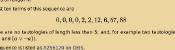Logic bot, pt. 2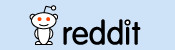How OEISbot worksRaspberry Pi weather station

Comments in green were written by me. Comments in blue were not written by me.
In part two you say a_{n+4} >= 2*a_n, and you have 13 years worth of tweets of length (say) 15-30. so there are 26 years worth length 19-34 characters, 13*2^n years worth of tweets of length between (15 + 4n) and (30 + 4n). In particular, setting n = 27, we have 13*2^{27} = 1744830464 years worth of tweets of length 123-138. I hope you have nice sturdy hardware!

Christian